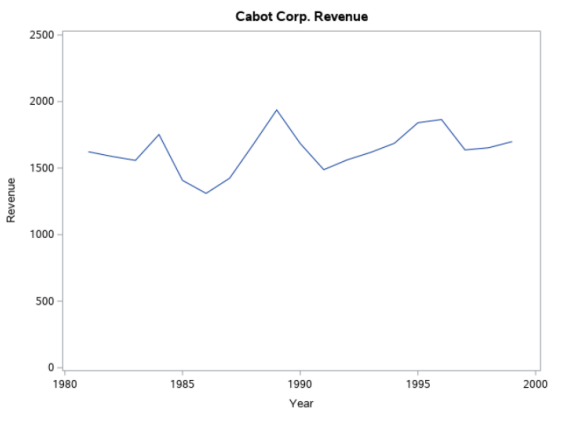# Moving Average

Let us consider the revenue from Cabot Corporation and see how we the Moving Average model can be useful to smooth the annual time series such as below.

By plotting the series, we can identify the factors affecting the movements. In the case of Cabot Corporation revenues, trend movement (long-term upward or downward) is obscured by the amount of variation from year to year.To obtain the better overall impression of the pattern in the series like above, we need to smooth the series.

Moving Average for a chosen period of length L consist of series of arithmetic means computed over a period of time containing data for length L.

Say we choose L = 3 then

MA(3) = (Y1 + Y2 + Y3) / 3 for the first three years

MA(3) = (Y2 + Y3 + Y4) / 3 for the first three years and so on.

Consider following sample data:

4.0, 5.0, 7.0, 6.0, 8.0, 9.0, 5.0, 2.0, 3.5, 5.5, 6.5

MA(3) = (4 + 5 + 7) / 3 = 5.33

Now, this 5.33 is centered on the middle value – the second value of the series.

MA(3) = (5 + 7 + 6) / 3 = 6 is centered on the middle value – the third value of the series.

MA(3) = (7 + 6 + 8) / 3 = 7 is centered on the middle value – the forth value of the series.

and so on.

By doing this, no moving averages can be obtained for first (L-1)/2 year and last (L-1)/2 year. So in our case, first 1 year and last year will be having missing value.

Data MA(3) MA(5)
4
5 5.33
7 6 6
6 7 7
8 7.66 7
9 7.33 6
5 5.33 5.5
2 3.5 5
3.5 3.66 4.5
5.5 5.16
6.5
The value of L is highly subjective. If dealing with annual time series data, L should be an odd number. As the L increases, smoothing will also increase but at the same time fewer number of moving averages will be computed.

Techinfoplace is a leading web solutions company that can provide you a complete range of products & solutions which help in improving your business by attracting more customers to raise your profits.

## Attended Events• Booth No.: E - 510
• EXHIBITION : 12 - 14 OCT 2017
• The Bombay Exhibition Centre at Goregaon East